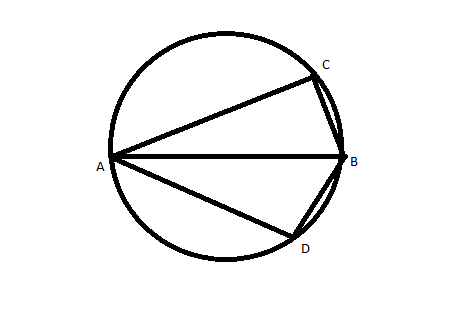Question 56

#AB is the diameter of the given circle, while points C and D lie on the circumference as shown. If AB is 15 cm, AC is 12 cm and BD is 9 cm, find the area of the quadrilateral ACBD.

Solution

Since ACBD is cyclic quadrilateral with diagonals as AB = 15 ad CD.
So area = $$\frac{1}{2} \times (AB) \times (CD)$$                  eq.(1)
Triangle ACB and ABD is right angled triangle
Let's say angle CBA = $$\theta$$
so $$CD = 2 \times (9 sin\theta)$$  (Where  $$sin\theta$$ = $$\frac{12}{15}$$)
CD = $$\frac{72}{5}$$
Putting values in eq. (1) , we will get area = 108 sq.cm.